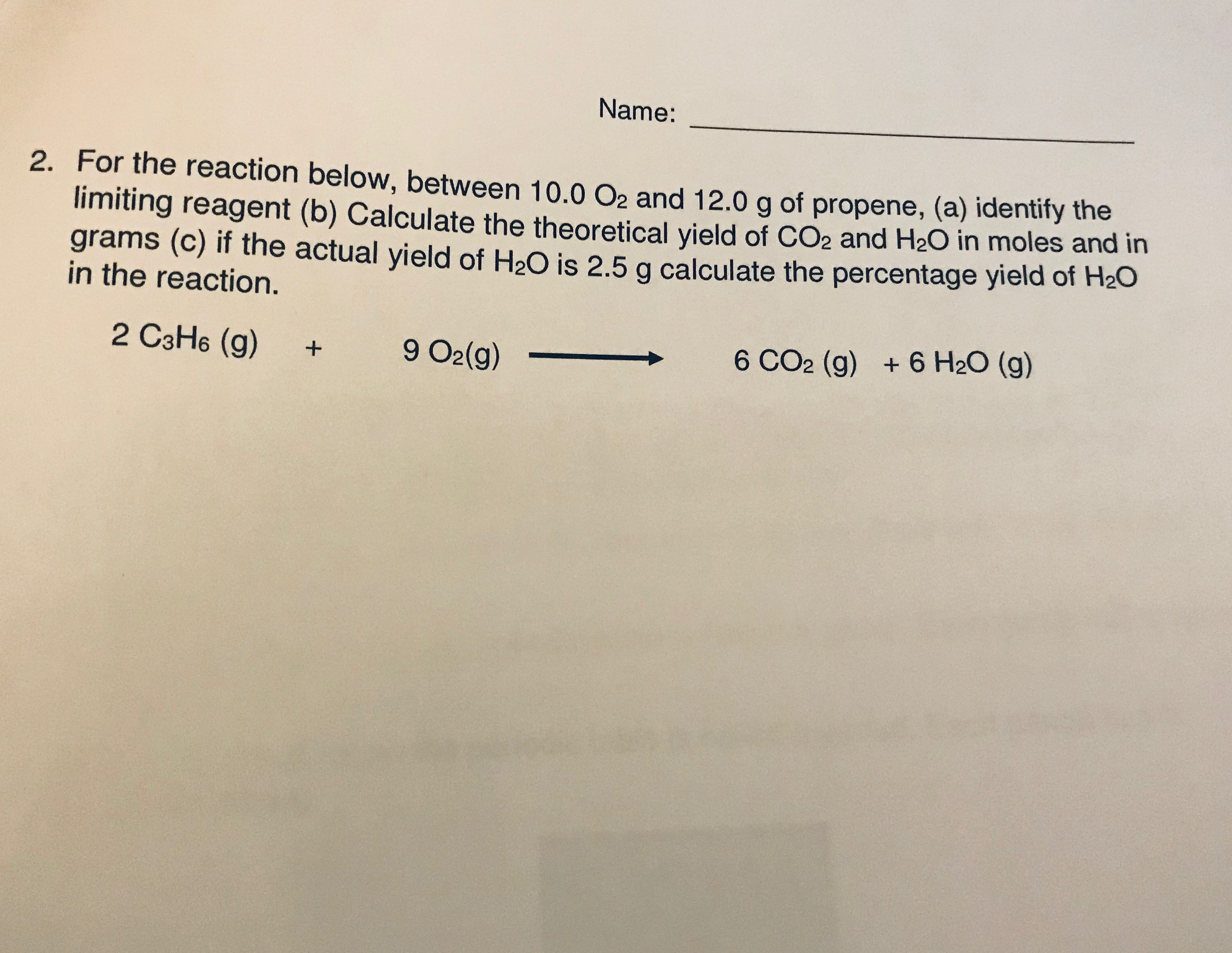# for the reaction below, between 10.0 O2 and 12.0g of propene, identify the limiting reagent, calculate the theoretical yield of CO2 and H2O in moles and in grams, if the actual yield of H2O is 2.5g calculate the percentage yield of H2O in the reaction 2C3H6 (g) + 9O2(g)------> 6CO2(g) + 6H2O(g)